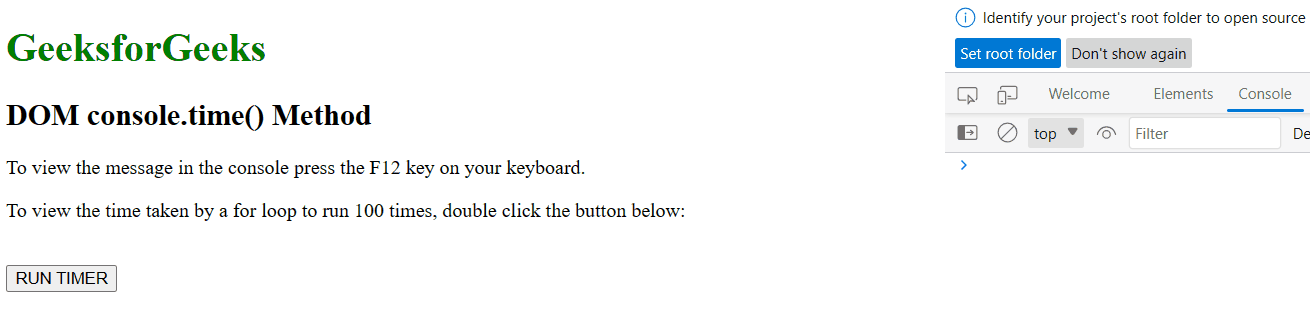# HTML DOM console time() Method

The console.time() method in HTML is used to start a timer in the console view. The console.time() method can be used for calculating the time of programs for various testing purposes. The label is sent as a parameter to the console.time() method

Syntax:

`console.time( label )`

Parameters: This method accepts a single parameter label which is optional and used to specify the label of the timer.

Example 1: Below programs illustrate the console.time() method in HTML.

## html

 `` `<``html``>` `  `  `<``head``>` `    ``<``title``>DOM console.time() Method in HTML` `    ``<``style``>` `        ``h1 {` `            ``color: green;` `        ``}` `    ``` ``   `<``body``>` `    ``<``h1``>GeeksforGeeks` `    ``<``h2``>DOM console.time() Method` `    ``<``p``>` `        ``To view the message in the console` `        ``press the F12 key on your keyboard.` `    ``` `    ``<``p``>` `        ``To view the time taken by a for loop` `        ``to run 100 times, double click the` `        ``button below:` `    ``` `    ``<``br``>` `    ``<``button` `ondblclick``=``"table_time()"``>` `        ``RUN TIMER` `    ``` `    ``<``script``>` `        ``function table_time() {` `            ``console.time();` `            ``for (i = 0; i < ``100``; i++) {` `                ``// Random code` `            ``}` `            ``console.timeEnd();` `        ``}` `    ``` `` `  `  ``

Output:Example 2: Calculate the time taken by a while loop using console.time() method

## HTML

 `` `<``html``>` `  `  `<``head``>` `    ``<``title``>DOM console.time() Method in HTML` `    ``<``style``>` `        ``h1 {` `            ``color: green;` `        ``}` `    ``` ``   `<``body``>` `    ``<``h1``>GeeksforGeeks` `    ``<``h2``>DOM console.time() Method` `    ``<``p``>` `        ``To view the message in the console` `        ``press the F12 key on your keyboard.` `    ``` `    ``<``p``>` `        ``To view the time taken by a for loop` `        ``to run 100 times, double click the` `        ``button below:` `    ``` `    ``<``br``>` `    ``<``button` `ondblclick``=``"table_time()"``>` `        ``RUN TIMER` `    ``` `    ``<``script``>` `        ``function table_time() {` `            ``i = 0;` `            ``console.time` `                ``("While loop for 100 iterations");` `            ``while (i < ``100``) {` `                ``i++;` `            ``}` `            ``console.timeEnd` `                ``("While loop for 100 iterations");` `        ``}` `    ``` `` `  `  ``

Output:Supported Browsers: The browser is supported by the console.time() Methods are listed below: